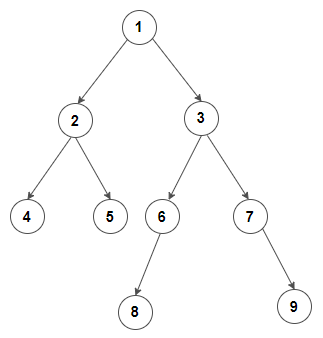# Find ancestors of given node in a Binary Tree

Given a binary tree, find all ancestors of given node in it.

For example, consider below binary treeAncestor of node 9 are 7, 3 and 1
Ancestor of node 6 are 3 and 1
Ancestor of node 5 are 2 and 1
. . .

The idea is to traverse the tree in postorder fashion and search for given node in the tree. If the node is found, we return true from the function. So for any node, if the given node is found in either its left subtree or its right subtree, then the current node is an ancestor of it.

C++ implementation –

The time complexity of above solution is O(n) and need O(h) extra space for the call stack where h is the height of the tree.

#### Iterative solution –

The idea is to main a map to store parent node information of all nodes present in the tree. Then we do a iterative pre-order traversal of the tree, and set parent pointer of each node. Finally, we print ancestors of given key by using parent map.

C++ implementation –

The time complexity of above solution is O(n) and auxiliary space used by the program is O(n).(1 votes, average: 5.00 out of 5)Loading...

Please use our online compiler to post code in comments. To contribute, get in touch with us.
Like us? Please spread the word and help us grow. Happy coding 🙂Subscribe
Notify ofGuest
Top Coder

nicely done..Guest

In recursive case, may be we could return if left returns true and not look in right subtree.Guest

why is 6 not a ancestor of the node 9..?Guest
Manish Sakariya

how can we return ancestor nodes as linked list?Guest
Shishir Ramesha

errr.. simple solution is to just do a binary search for the given node and print the values along the path ?!!Guest

You can’t do binary search on a binary tree. If you mean perform standard search operation, please note that the tree in question is binary tree, and not a binary search tree.